# Circles - Using π - Level 1

## Practise using pi to calculate various circle measurements.

##### MenuLevel 1Level 2Level 3Level 4Level 5Level 6ExamHelpMore

This is level 1; find the circumference given the radius or diameter. Give your answers correct to three significant figures. You can earn a trophy if you get at least 7 correct. The diagrams are not drawn to scale.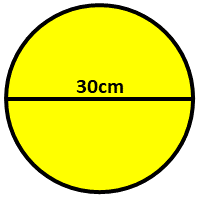The diameter of this circle is 30cm.Calculate the distance around the circle (circumference).Don't forget to round off your answer to three significant figures. cm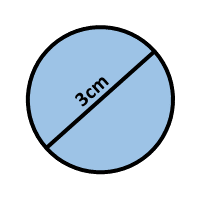Calculate the circumference of this circle if the diameter is 3cm. cm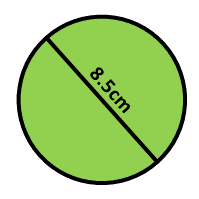This third circle has a diameter of 8.5cm.Calculate its circumference. cm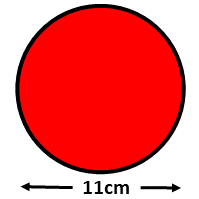You should be getting good at finding the circumference of a circle by now.Calculate the circumference of this circle if the diameter is 11cm. cm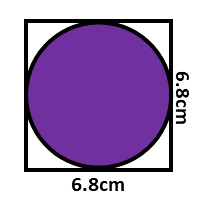Calculate the circumference of this circle drawn inside a square with sides of length 6.8cm. cmYou are given the radius of this circle, not the diameter. What is its circumference? cm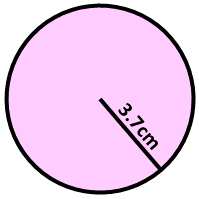Calculate the circumference of a circle if the radius is 3.7cm. cm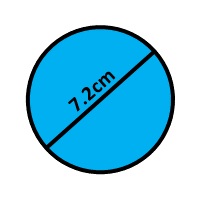Calculate the circumference of a circle if the diameter is 7.2cm. cmCalculate the circumference of circular jar if its radius is 58mm. mm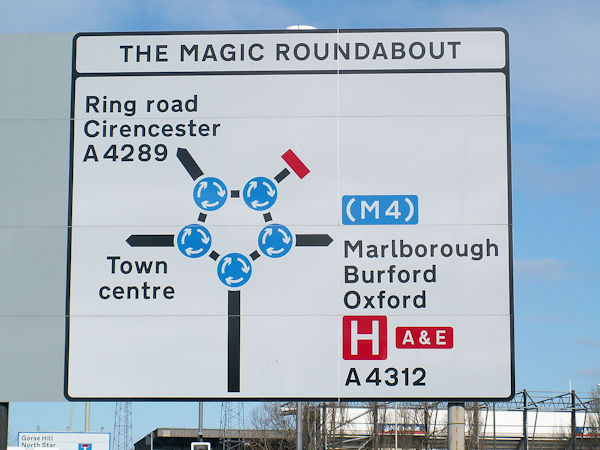The magic roundabout in Swindon consists of five small traffic islands around one large island.Vehicles can drive clockwise around the small islands and anticlockwise around the large island.If a car drives around every island once and finishes in the same place it starter work out the total distance travelled.Assume the small islands have a radius of 8.8m and the large island has a radius of 26.4m. mCheck

This is Circles - Using pi level 1. You can also try:
Level 2 Level 3 Level 4 Level 5 Level 6 Composites

## Instructions

Try your best to answer the questions above. Type your answers into the boxes provided leaving no spaces. As you work through the exercise regularly click the "check" button. If you have any wrong answers, do your best to do corrections but if there is anything you don't understand, please ask your teacher for help.

When you have got all of the questions correct you may want to print out this page and paste it into your exercise book. If you keep your work in an ePortfolio you could take a screen shot of your answers and paste that into your Maths file.

## Transum.org

This web site contains over a thousand free mathematical activities for teachers and pupils. Click here to go to the main page which links to all of the resources available.

Please contact me if you have any suggestions or questions.## More Activities:

Mathematicians are not the people who find Maths easy; they are the people who enjoy how mystifying, puzzling and hard it is. Are you a mathematician?

Comment recorded on the 24 May 'Starter of the Day' page by Ruth Seward, Hagley Park Sports College:

"Find the starters wonderful; students enjoy them and often want to use the idea generated by the starter in other parts of the lesson. Keep up the good work"

Comment recorded on the 1 August 'Starter of the Day' page by Peter Wright, St Joseph's College:

"Love using the Starter of the Day activities to get the students into Maths mode at the beginning of a lesson. Lots of interesting discussions and questions have arisen out of the activities.
Thanks for such a great resource!"

Each month a newsletter is published containing details of the new additions to the Transum website and a new puzzle of the month.

The newsletter is then duplicated as a podcast which is available on the major delivery networks. You can listen to the podcast while you are commuting, exercising or relaxing.

Transum breaking news is available on Twitter @Transum and if that's not enough there is also a Transum Facebook page.

#### Number Crunch Saga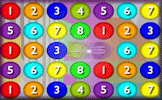A lively numeracy game requiring you to align three numbers to create the given target sum or product. There are five levels to this online game and a virtual Transum Trophy available for each level.

There are answers to this exercise but they are available in this space to teachers, tutors and parents who have logged in to their Transum subscription on this computer.

A Transum subscription unlocks the answers to the online exercises, quizzes and puzzles. It also provides the teacher with access to quality external links on each of the Transum Topic pages and the facility to add to the collection themselves.

Subscribers can manage class lists, lesson plans and assessment data in the Class Admin application and have access to reports of the Transum Trophies earned by class members.

If you would like to enjoy ad-free access to the thousands of Transum resources, receive our monthly newsletter, unlock the printable worksheets and see our Maths Lesson Finishers then sign up for a subscription now:

Subscribe

## Go Maths

Learning and understanding Mathematics, at every level, requires learner engagement. Mathematics is not a spectator sport. Sometimes traditional teaching fails to actively involve students. One way to address the problem is through the use of interactive activities and this web site provides many of those. The Go Maths page is an alphabetical list of free activities designed for students in Secondary/High school.

## Maths Map

Are you looking for something specific? An exercise to supplement the topic you are studying at school at the moment perhaps. Navigate using our Maths Map to find exercises, puzzles and Maths lesson starters grouped by topic.

## Teachers

If you found this activity useful don't forget to record it in your scheme of work or learning management system. The short URL, ready to be copied and pasted, is as follows:

Alternatively, if you use Google Classroom, all you have to do is click on the green icon below in order to add this activity to one of your classes.

It may be worth remembering that if Transum.org should go offline for whatever reason, there are mirror sites at Transum.com and Transum.info that contain most of the resources that are available here on Transum.org.

When planning to use technology in your lesson always have a plan B!

Wednesday, January 9, 2019

Do you have any comments? It is always useful to receive feedback and helps make this free resource even more useful for those learning Mathematics anywhere in the world. Click here to enter your comments.For All:

## Description of Levels

CloseLevel 1 - find the circumference given the radius or diameter.

Level 2 - find the radius or diameter given the circumference.

Level 3 - find the area of a circle given either the radius or diameter.

Level 4 - the areas of circles are given, find either the radius, diameter or circumference.

Level 5 - the radius and angle subtended at the centre of the circle are given, find the length of the arc or area of the sector of the circle.

Level 6 - this level has mixed questions about the circle. Most of these questions will require a multi-part calculation once the situation described in the question has been understood.

Areas of composite shapes requires an ability to calculate the areas of other shapes such as rectangles, triangles and trapezia.

Exam Style questions are in the style of GCSE or IB/A-level exam paper questions and worked solutions are available for Transum subscribers.

Answers to this exercise are available lower down this page when you are logged in to your Transum account. If you don’t yet have a Transum subscription one can be very quickly set up if you are a teacher, tutor or parent.

## Help with circle calculations

Use a calculator for this exercise. All of the calculations you will do involve the number π (pronounced pi) which is roughly equal to 3.141592. You should use the π button on your calculator to get this number into your calculation.

Let r be the radius, d the diameter, C the circumference and A the area of a circle.

C = πd    [i.e., to find the circumference multiply the length of the diameter by pi]

A = πr2    [i.e., to find the area multiply the square of the radius by pi]For arcs multiply the circumference by the angle subtended at the centre and divide by 360.

For sector area multiply the circle area by the angle subtended at the centre and divide by 360.

For help using a calculator with circle calculations see Calculator Workout.

For more on this topic see our Circles page.

Answers to this exercise are available lower down this page when you are logged in to your Transum account. If you don’t yet have a Transum subscription one can be very quickly set up if you are a teacher, tutor or parent.

Close